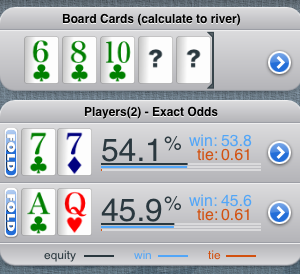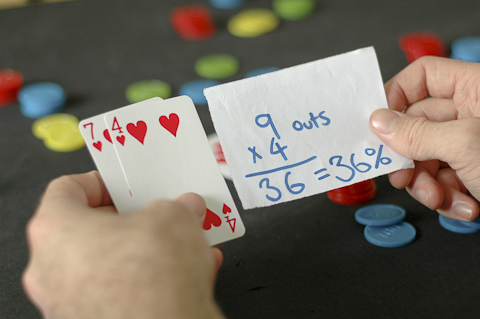top corner

# How to Quickly Calculate Hand Odds in PokerIt’s no secret that the game of poker has become an increasingly mathematical one. Many modern-day poker players use at least some mathematical concepts when playing live or online poker. However, what about those of us that aren’t quite as adept in math?

One common question we’re asked is how to figure out hand odds when playing Texas Hold’em. How can you know the odds of making a winning hand on the flop? The math behind it can be a bit complicated, but we have a simple solution. It is known as the rule of 4 and 2.

Phil Gordon came up with his simple method of figuring out hand odds on the flop and turn. While the numbers are not pinpoint exact, it is close enough. Today we will tell you how to figure out hand odds using the rule of 4 and 2.

## Figuring Out Odds on the Flop

Once you get to the flop, you can quickly figure out your odds of making a winning hand by the river. First, you need to figure out how many outs will give you a likely winning hand. For example, if you have A-K and the flop falls 2s-5c-9d, it’s likely that an ace or king will give you the best hand by the river.

As such, you have six outs that can give you a winning hand. Take these six and multiply at by four. In this case, you will come up with 24. Next, add 2 to that number. This gives you 26. You have around a 26 percent chance of making a winning hand by the river.

Naturally, the more outs you have, the higher your odds. Let’s take a hand such as Ks-10s and the flop falls Qs-Jd-4s. In this case, you have a straight draw and a flush draw. You have nine outs to a flush and eight outs to a straight. You may also win if you hit a king. This gives you 20 outs.

Taking 20 outs and multiplying it by four, you get 60. Add two and get 62 to reach a 62 percent chance that you will make a winning hand by the river.## Figuring Out Odds on the Turn

On the turn, you will use a similar process, but different calculations. Take our initial example of A-K. You will take your outs and multiply it by two. This gives you 16. Next, add 1 to the total. This means you have a 17 percent chance to hit your hand by the river.

For our second example with 20 outs, you will have a 41 percent chance to hit your hand on the river. Of course, you have to pay close attention to the turn card to make sure that all of your outs are still live. If you have a hand like K-J on a low flop and an ace hits the turn, kings may not improve your hand.

## Why Are Hand Odds Important

Hand odds are important because they are the first step in calculating pot odds. We will cover pot odds more in-depth in a future article. However, the general concept is that if your hand odds are greater than your pot odds, you are safe to continue in the hand.

For now, it is important that you get familiar with learning how to properly calculate your odds. As stated earlier, the rule of 4 and 2 is not exact, however, the percentages will only be off by a couple of percents. This difference is seldom enough to make a difference in your decision making. Once you have mastered hand odds, you can move on to apply this knowledge to pot odds.

bottom corner